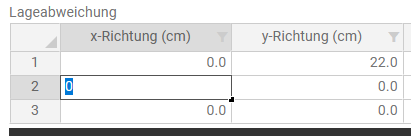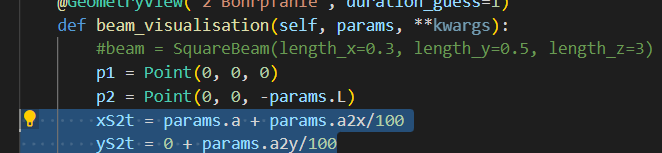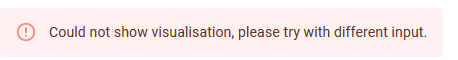# Set values from a table as input parameter

Hi

I need to calculate a few parameters:
Length_A = 1 + A
Length_B = 1 + B
Length_C = 1 + C
I now want, that the user can input the A, B and C in a Table, like this:Unfortunately I don’t know, how I can access to the table values to set the value as a parameter?

Hi Manuel,

Welcome to the community forum, thanks for posting!

The answer to your question depends on what you are going to use the parameter for. If you want to use the values in the table for a calculation or a view, you can simply access them in your `Controller` class using the `params`. So if you’d want to use the values, as you say, to add 1 to each and present them in a `DataView` for example, your app would look something like this:

``````class Parametrization(ViktorParametrization):
my_table = Table("My table")
my_table.x_direction = NumberField("x_direction", suffix='cm')

class Controller(ViktorController):
label = 'My Entity Type'
parametrization = Parametrization

@DataView("Calculation output", duration_guess=1)
def my_calculation(self, params, **kwargs):

x_values = [row["x_direction"] for row in params.my_table]

data_groups = []
for i, val in enumerate(x_values):
if val:
data_groups.append(
DataGroup(DataItem(f'Data item {i + 1}', val + 1))
)

data = DataGroup.from_data_groups(data_groups)

return DataResult(data)
``````

Here you can see that the values from the table are used with the line `x_values = [row["x_direction"] for row in params.my_table]`. The table in the params is processed as a list with a dictionary-type value for each row in the table.

If on the other hand you’d want to present the adjusted (+1) value of the entries in the table to the user in the parametrization itself, you would have to utilize a `SetParamsButton`. If you need more help using that, let me know!

Has that answered your question? If not, please provide a little more context to how you want to use the values from your table.

Many thanks to your reply. It already clears a little bit how to use tables input values.

In my object, I would like to define a parametrized 3D column along a line (CircularExtrusion):
p1 = Point(0, 0, 0)
p2 = Point(0, 0, params.L)
line1 = Line(p1, p2)
column = CircularExtrusion(params.D, linie1)

But now I want to set the Params.L with a calcution based on the table input values:

Length of the pile = params.L + Value of the table.

For example Table:
row 1, x-Direction has the value = 5
row 2, x-Direction has the value = 10

First Colum: params.L + 5 is the new length of the column
Second Colum: params.L + 10 is the new length of the column

So I would need to set the values from the table into a calculation for point definitions.

Hi

I would also need to know, how can I calculate with tangens functions?

The vertical deviation of a column for example is 2°. This means that the bottom of the pile is:
Tangens(2°) * L
Do I need to add: math.tan(2) ?

And can anyone tell me, why it is not possible to set new variables in the class Parametrization() set?

Because of the reason this is not possible. I always need to set the variables new in every sheet.
For the 3D view:And for the next 3d view:

:

Hi Manuel,

By design, VIKTOR’s call flow is (mostly) stateless, this means that in principle nothing is saved besides the input itself, and the functions to get to the result. The reasoning for this is that we believe that the same input from the user should always return the same result, no matter in what order you see the views. You can find more information about this here.

I think that the variables that you are using such as the vertical deviation are not meant to be user input, but rather intermediary results for the developer? In that case they will indeed be recalculated every time. This can be a bit counter intuitive at first, but it guarantees the statelessness.

I totally understand that you do not want to rewrite all the variables every time, therefore I usually make a model class to make it easier for myself. I use this model to put all my calculations in, and use this model for every view. in your case it might look something like the example below.

As the calculations are fast, it should not have an effect on the user experience that you are calculating the same cosine twice. However, once you start to run large analyses, you might want to look into memoization and storage to avoid full statelessness and store intermediary results.

I hope this helps.

Paulien

``````import math
from dataclasses import dataclass

# c, L a2x and a2y are user input,
# xS2t, yS2t and bottom_of_pile are calculated
@dataclass
class Pile:
a: float
L: float
a2x: float
a2y: float

def xS2t(self):
return self.a + self.a2x/100

def yS2t(self):
return self.a2x/100

def bottom_of_pile(self):
return self.L * math.tan(2)

def p2(self):
return Point(0, 0, self.L)
``````

``````    @DataView("Calculation output", duration_guess=1)
def my_calculation(self, params, **kwargs):
piles = []
# create one pile object for each row in the table
for row in params.table:
piles.append(Pile(row["a"], row["L"], row["a2x"], row["a2y"]))

data_groups = []
# show the intermediate results for each pile to user
for i, pile in enumerate(piles):
data_groups.append(
DataGroup(
DataItem('Pile', i+1),
DataItem('length', pile.L),
DataItem('bottom of pile', pile.bottom_of_pile()),
)
)

data = DataGroup.from_data_groups(data_groups)
return DataResult(data)

@WebView("Other Calculation output", duration_guess=1)
def my_other_calculation(self, params, **kwargs):
pile = Pile(1, 2, 3, 4)
return WebResult(html=f"A pile with a={pile.a}, L={pile.L}, a2x={pile.a2x} "
f"and a2y= {pile.a2y} will have yS2t = {pile.yS2t()}")
``````

edit: changed column to pile so not to be confusing with table rows and columns

Hi

Thanks for your explanation. Your are using an iteration function (for i, column…)
I am still confused how to catch a single value of a table. For example I want to catch the valueof row 2 / column3:

What Code do I need for the value 2.0 to get this one as user input for simple calculations?

Like in your Code, the Values `a2x and a2y` are inputs from the table. But you are not catching this values from the input table

Hi

I was now trying to implement your code. But there is only an Error coming in both Views you created:Hi Manuel,

I do not know how you have called your parameters, so I had to make an assumption, I edited the code so the second view always works no matter how you structured the input.

I go through the table here:

``````for row in params.table:  # or params.yourtabname.yourtablename
# assuming the table has 4 columns called a, L, a2x and a2y
piles.append(Pile(row["a"], row["L"], row["a2x"], row["a2y"]))
``````

If you have the following parametrization:

``````class ProjectParametrization(Parametrization):
yourtabname = Tab("tab name")
yourtabname.yourtablename = Table("Lageabwichung")
yourtabname.yourtablename.x = NumberField("x-richtung")
yourtabname.yourtablename.y = NumberField("y-richtung")
yourtabname.yourtablename.neigung_x = NumberField("Neigung x richtung")
``````

You can acces the highlighted value with:

``````second_row = params.yourtabname.yourtablename # is a dictionary with keys x, y and neigung_x
selected_value = second_row["neigung_x"]
# or
params.yourtabname.yourtablename["neigung_x"]
``````

kind regards,
Paulien